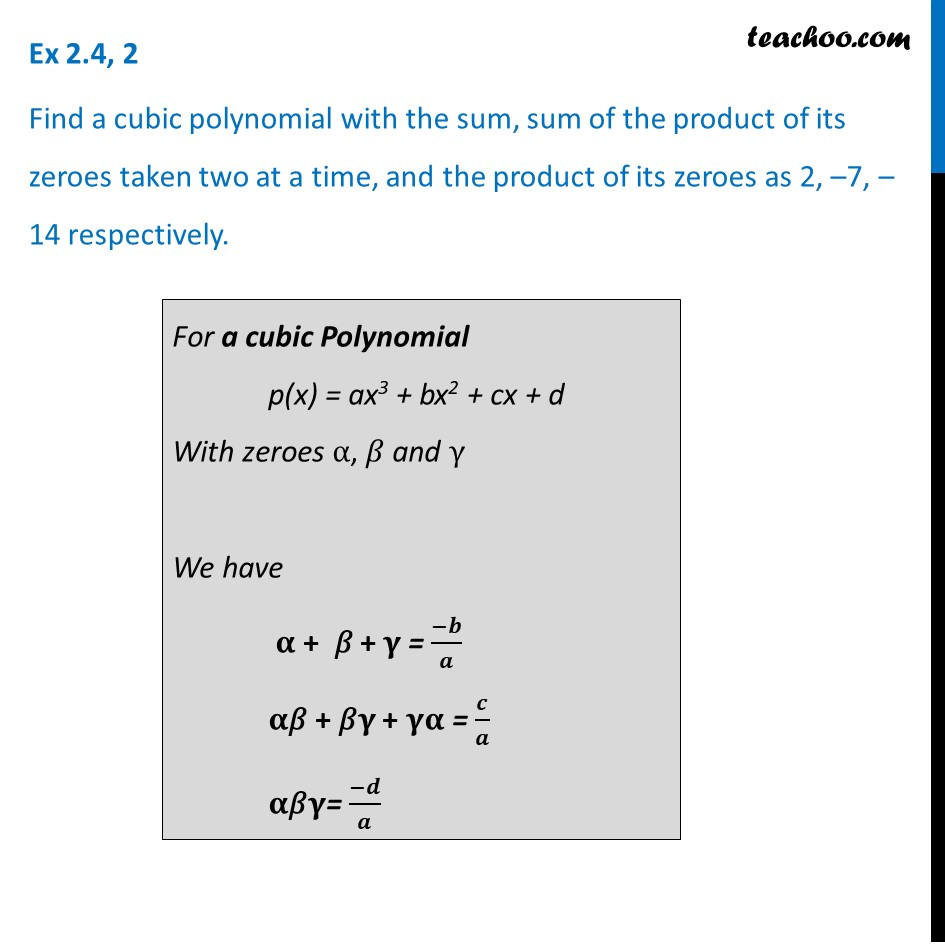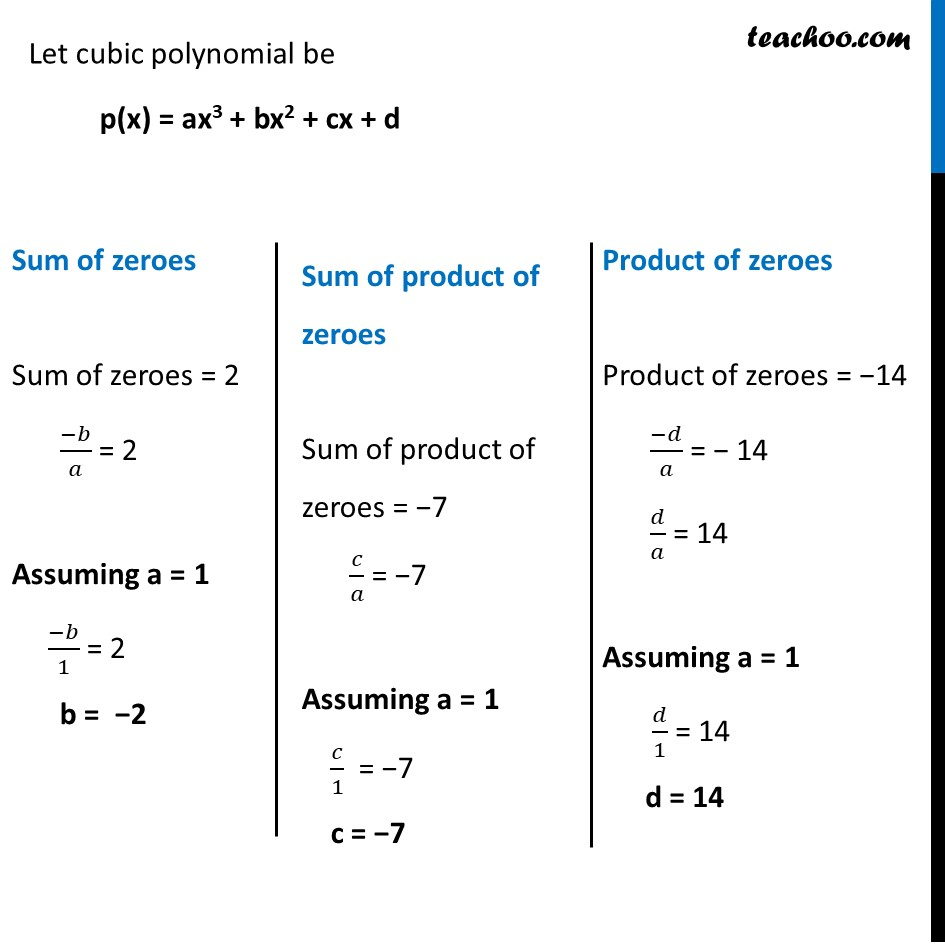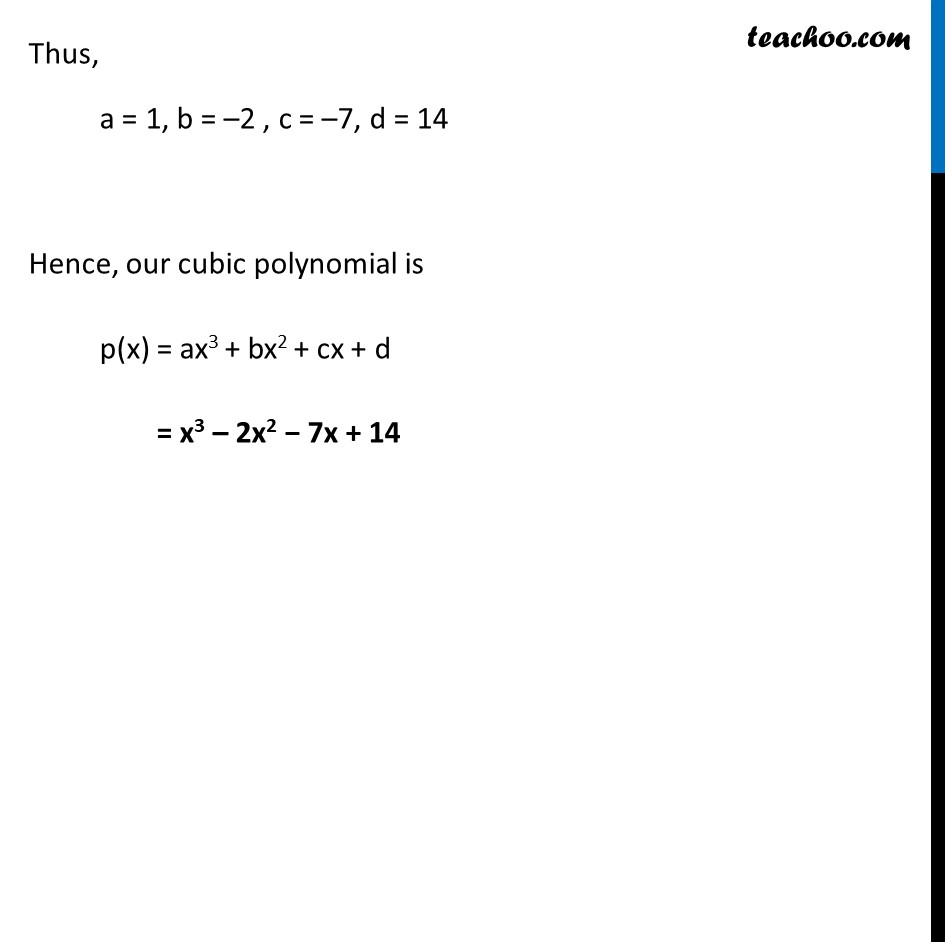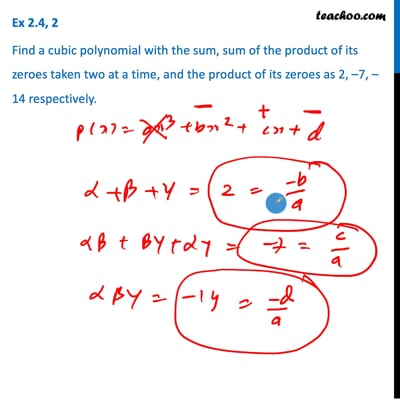Ex 2.4 (Optional)

Chapter 2 Class 10 Polynomials
Serial order wiseThis video is only available for Teachoo black users

Maths Crash Course - Live lectures + all videos + Real time Doubt solving!

### Transcript

Ex 2.4, 2 Find a cubic polynomial with the sum, sum of the product of its zeroes taken two at a time, and the product of its zeroes as 2, –7, –14 respectively. For a cubic Polynomial p(x) = ax3 + bx2 + cx + d With zeroes α, 𝛽 and γ We have 𝛂 + 𝛽 + 𝛄 = (−𝒃)/𝒂 𝛂"𝛽" + 𝛽𝛄 + 𝛄𝛂 = 𝒄/𝒂 𝛂"𝛽" 𝛄= (−𝒅)/𝒂 Let cubic polynomial be p(x) = ax3 + bx2 + cx + d Sum of zeroes Sum of zeroes = 2 (−𝑏)/𝑎 = 2 Assuming a = 1 (−𝑏)/1 = 2 b = −2 Sum of product of zeroes Sum of product of zeroes = −7 𝑐/𝑎 = −7 Assuming a = 1 𝑐/1 = −7 c = −7 Product of zeroes Product of zeroes = −14 (−𝑑)/𝑎 = − 14 𝑑/𝑎 = 14 Assuming a = 1 𝑑/1 = 14 d = 14 Thus, a = 1, b = –2 , c = –7, d = 14 Hence, our cubic polynomial is p(x) = ax3 + bx2 + cx + d = x3 – 2x2 − 7x + 14